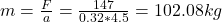A bicycle wheel has a radius R = 32.0 cm and a mass M = 1.82 kg which you may assume to be concentrated on the outside radius. A resistive f

Question

A bicycle wheel has a radius R = 32.0 cm and a mass M = 1.82 kg which you may assume to be concentrated on the outside radius. A resistive force f =  N (due to the ground) is applied to the rim of the tire. A force F is applied to the sprocket at radius r such that the wheel has an angular acceleration of α = 4.50 rad/s2 . The tire does not slip.(a) If the sprocket radius is 4.53 cm, what is the force F (N)?(b) If the sprocket radius is 2.88 cm, what is the force F (N)?(c) What is the combined mass of the bicycle and rider (kg)?

in progress 0
6 months 2021-07-19T16:27:54+00:00 1 Answers 15 views 0

a) The force is 1056.93 N

b) If the sprocket radius is 2.88 cm, the force is 1662.47 N

c) The combined mass is 102.08 kg

Explanation:

a) The net torque is: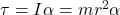Where

m = 1.82 kg

r = 32 cm = 0.32 m

Replacing: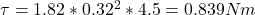The force is: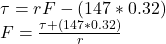Where

r = 4.53 cm = 0.0453 m

Replacing: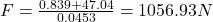b) If r = 2.88 cm = 0.0288 m, replacing in the previous equation: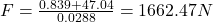c) The combined mass is: